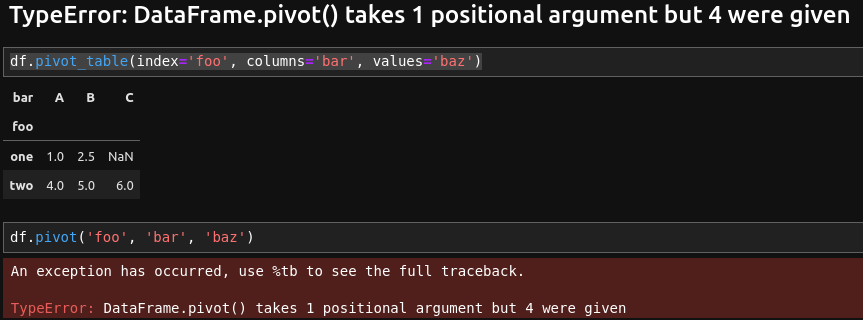In this tutorial, we'll take a closer look at the Pandas error, TypeError: DataFrame.pivot() takes 1 positional argument but 4 were given - Pandas. First, we'll create an example of how to produce it. Next, we'll explain the leading cause of the exception. And finally, we'll see how to fix it.

## Example

Let's have a DataFrame like:

``````import pandas as pd
df = pd.DataFrame({'foo': ['one', 'one', 'one', 'two', 'two', 'two'],
'bar': ['A', 'B', 'B', 'A', 'B', 'C'],
'baz': [1, 2, 3, 4, 5, 6],
'zoo': ['x', 'y', 'z', 'q', 'w', 't']})

``````

with data:

foo bar baz zoo
0 one A 1 x
1 one B 2 y
2 one B 3 z
3 two A 4 q
4 two B 5 w

When running a code like the one below:

``````df.pivot('foo', 'bar', 'baz')
``````

we get error message: TypeError: DataFrame.pivot() takes 1 positional argument but 4 were given## Cause

The reason for the error is that:

all arguments of DataFrame.pivot are keyword-only.

Which means that we need to provide argument names for each argument passed to this method.

You can read more on this link: ENH: Keep positional arguments for pivot #51359

The code above was working in the past. Pandas community is doing efforts to make Pandas code more:

• explicit

## Solution

To solve error - TypeError: DataFrame.pivot() takes 1 positional argument but 4 were given - we need to provide all arguments by name:

``````df.pivot_table(index='foo', columns='bar', values='baz')
``````

This code will work fine.

## Conclusion

We've explained Pandas's TypeError: DataFrame.pivot() takes 1 positional argument but 4 were given error. Then, we discussed the reason and shared a discussion on the topic. Lastly, we discussed how to resolve the error.